# Colleges with the lowest SAT scores in Mississippi

Top 8 colleges in Mississippi with the lowest SAT scores
Looking for the colleges with the lowest SAT scores in Mississippi? Well you're in luck! We've compiled a national college database and have created a list of the top 8 universities with the lowest SAT scores in Mississippi below. If you are not a good test taker or worried about your test scores, this list is for you. These are the schools whose applicants had the lowest average SAT scores in Mississippi, which means that you can get into these colleges with a lower SAT score. We also include each college's ACT scores and acceptance rate so that you can see where you would have the easiest time getting in. Read on to find out more.

## Jackson State University SAT scores

The average SAT score for Jackson State University is 930.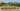The average SAT score of 930 breaks down into:

• SAT math: 465

The average ACT score for Jackson State University is 17 and their acceptance rate is 68.9%.

## Alcorn State University SAT scores

The average SAT score for Alcorn State University is 970.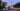The average SAT score of 970 breaks down into:

• SAT math: 490

The average ACT score for Alcorn State University is 19 and their acceptance rate is 78.6%.

## Delta State University SAT scores

The average SAT score for Delta State University is 1067.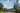The average SAT score of 1067 breaks down into:

• SAT math: 540

The average ACT score for Delta State University is 21 and their acceptance rate is 85%.

## University of Southern Mississippi SAT scores

The average SAT score for University of Southern Mississippi is 1070.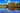The average SAT score of 1070 breaks down into:

• SAT math: 530

The average ACT score for University of Southern Mississippi is 23 and their acceptance rate is 98.5%.

## William Carey University SAT scores

The average SAT score for William Carey University is 1090.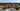The average SAT score of 1090 breaks down into:

• SAT math: 590

The average ACT score for William Carey University is 25 and their acceptance rate is 93%.

## Belhaven University SAT scores

The average SAT score for Belhaven University is 1123.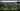The average SAT score of 1123 breaks down into:

• SAT math: 550

The average ACT score for Belhaven University is 22 and their acceptance rate is 59.1%.

## The University of Mississippi SAT scores

The average SAT score for The University of Mississippi is 1160.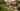The average SAT score of 1160 breaks down into:

• SAT math: 575

The average ACT score for The University of Mississippi is 25 and their acceptance rate is 88.1%.

## Mississippi State University SAT scores

The average SAT score for Mississippi State University is 1180.The average SAT score of 1180 breaks down into:

• SAT math: 580